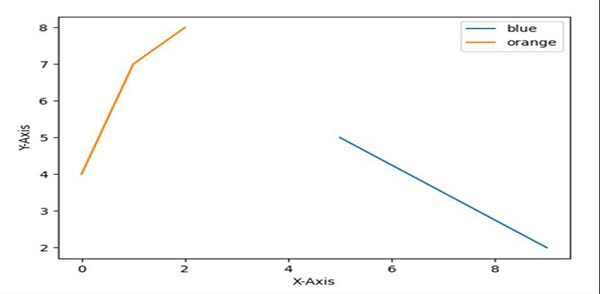# Show only certain items in legend Python Matplotlib

PythonMatplotlibServer Side ProgrammingProgramming

Using plt.legend(), we can add or show certain items just by putting the values in the list.

## Steps

• Set the X-axis label using plt.xlabel() method.

• Set the Y-axis label using plt.ylabel() method.

• Plot the lines using the lists that are passed in the plot() method argument.

• Location and legend_drawn flags can help to find a location and make the flag True for border.

• Set the legend with “blue” and “orange” elements.

• To show the figure use plt.show() method.

## Example

import matplotlib.pyplot as plt

plt.ylabel("Y-axis ")
plt.xlabel("X-axis ")

plt.plot([9, 5], [2, 5], [4, 7, 8])

location = 0 # For the best location
legend_drawn_flag = True
plt.legend(["blue", "orange"], loc=0, frameon=legend_drawn_flag)

plt.show()

## Output